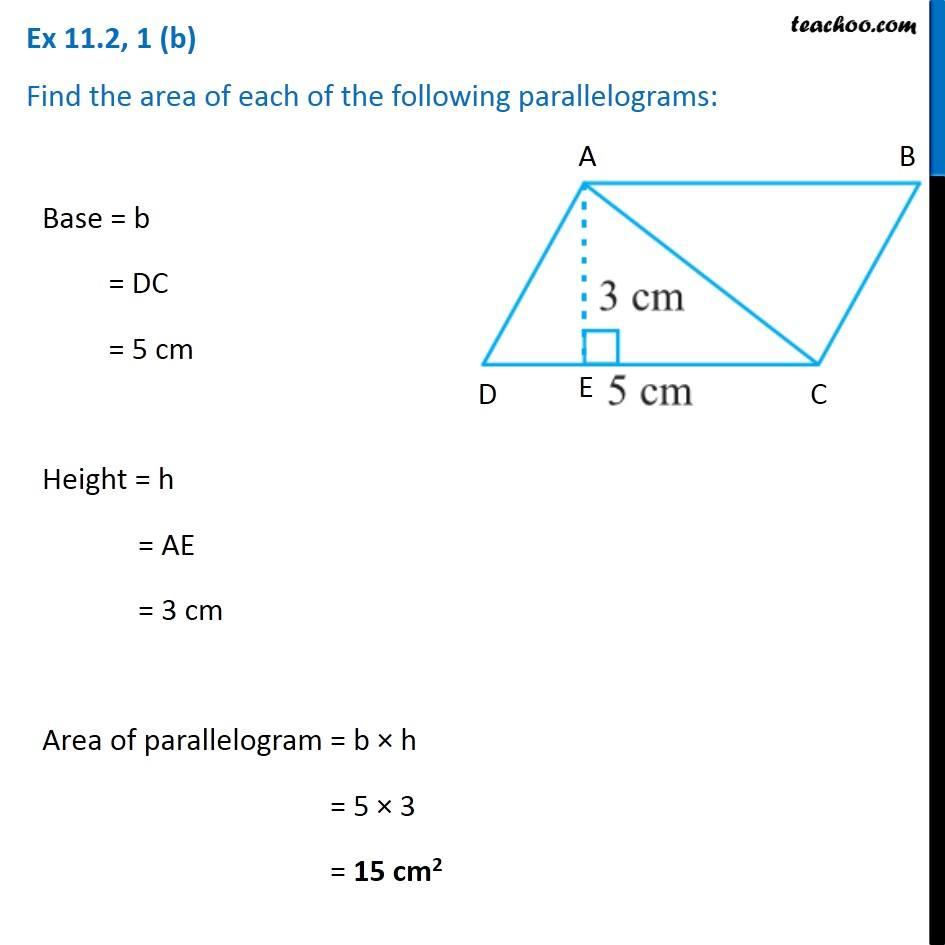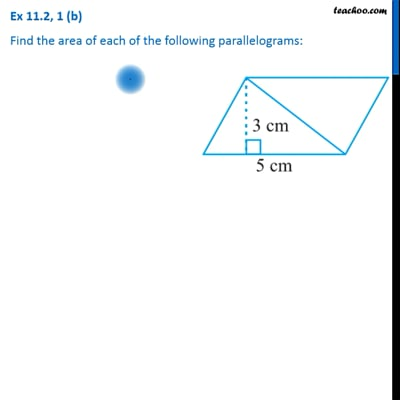Ex 11.2

Chapter 11 Class 7 Perimeter and Area
Serial order wiseThis video is only available for Teachoo black users

Get live Maths 1-on-1 Classs - Class 6 to 12

### Transcript

Ex 11.2, 1 (b) Find the area of each of the following parallelograms: Base = b = DC = 5 cm Height = h = AE = 3 cm Area of parallelogram = b × h = 5 × 3 = 15 cm2